# Precalculus : Understand features of hyperbolas and ellipses

## Example Questions

1 2 5 6 7 8 9 10 11 13 Next →

### Example Question #213 : Conic Sections

Which point is one of the foci of the hyperbola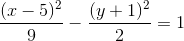?

Possible Answers: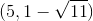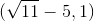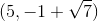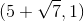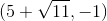Correct answer:Explanation:

To find the foci of a hyperbola, first determine a and b, and then use the relationship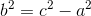In this case, the major axis is horizontal since x comes first, so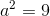and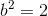.

Solve for c: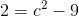add 9 to both sides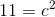take the square root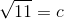Since the center is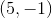and the major axis is the horizontal one, our foci are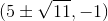. The only choice that works is.

### Example Question #214 : Conic Sections

Determine the length of the foci for the following hyperbola equation: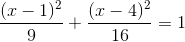Possible Answers: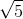Correct answer:Explanation:To solve, simply use the follow equation where c is the length of the foci.

In this particular case,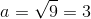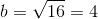Thus,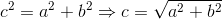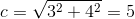### Example Question #215 : Conic Sections

Find the foci of the hyperbola with the following equation: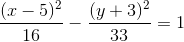Possible Answers: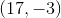and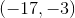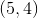and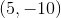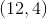and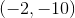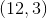and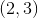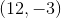and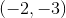Correct answer:andExplanation:

The standard form of the equation for a hyperbola is given by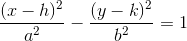The foci are located at (h+c, k) and (h-c, k), where c is found by using the formula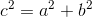Since our equation is already in standard form, you can see that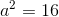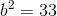Plugging into the formula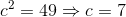So the foci are found at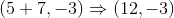AND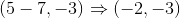### Example Question #216 : Conic Sections

How can this graph be changed to be the graph of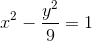?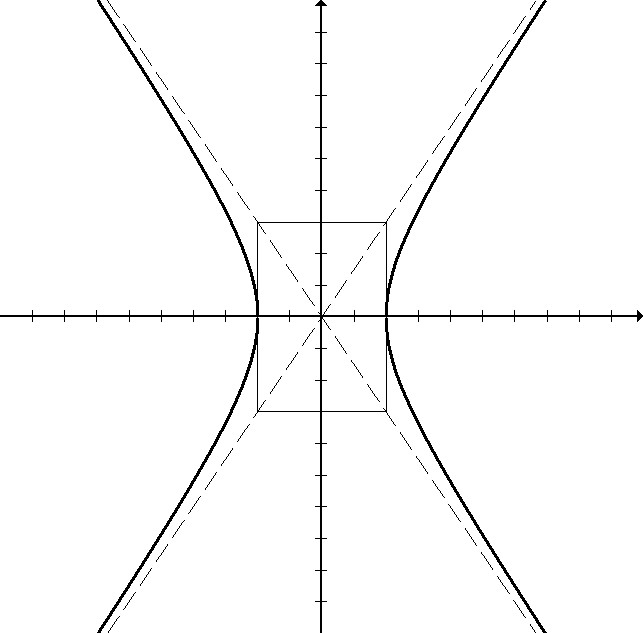Possible Answers:

The graph should be an ellipse and not a hyperbola.

The center box should extend up toand down to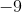, stretching the graph.

The-intercepts should be at the points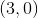and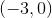.

The graph should have-intercepts and not-intercepts.

The-intercepts should be at the points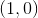and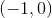.

Correct answer:

The-intercepts should be at the pointsand.

Explanation:

This equation should be thought of as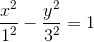.

This means that the hyperbola will be determined by a box with x-intercepts at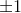and y-intercepts at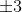.

The hyperbola was incorrectly drawn with the intercepts at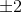instead.

### Example Question #217 : Conic Sections

Which of the following would NOT be true of the graph for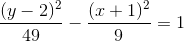?

Possible Answers:

All of these statements are true.

The graph never intersects with the-axis.

The graph opens up and down.

The graph is centered at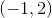.

The graph never intersects with the-axis.

Correct answer:

The graph never intersects with the-axis.

Explanation:

The graph should look like this: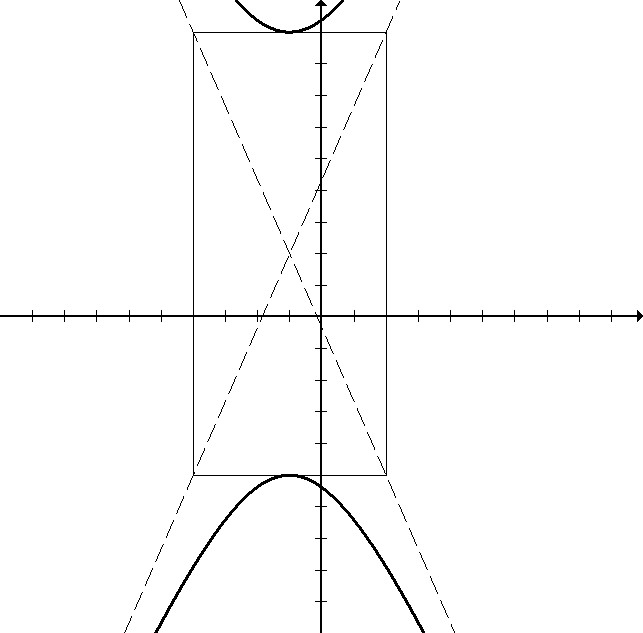### Example Question #121 : Hyperbolas And Ellipses

Which of these equations produce this graph, rotated 90 degrees?Possible Answers: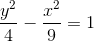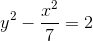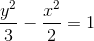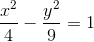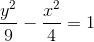Correct answer:Explanation:

Rotated 90 degrees, this graph would be opening up and down instead of left and right, so the equation will have the y term minus the x term.

The box that the hyperbola is drawn around will also rotate. It will now be up/down 2 and left/right 3.

This makes the correct equation.

### Example Question #219 : Conic Sections

What is the equation of the conic section graphed below?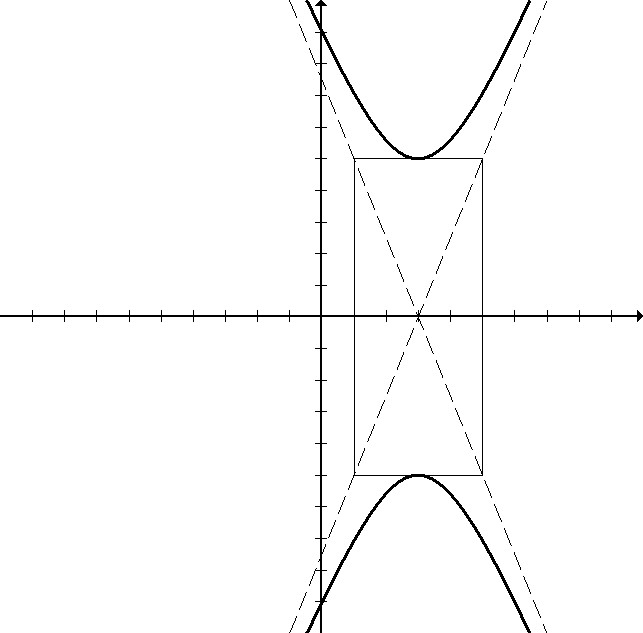Possible Answers: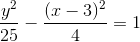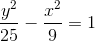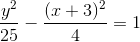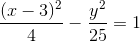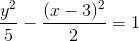Correct answer:Explanation:

The hyperbola pictured is centered at, meaning that the equation has a horizontal shift. The equation must have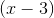rather than just x. The hyperbola opens up and down, so the equation must be the y term minus the x term. The hyperbola is drawn according to the box going up/down 5 and left/right 2, so the y term must beor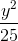, and the x term must be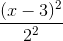or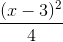.

1 2 5 6 7 8 9 10 11 13 Next →

### All Precalculus Resources